MATHEMATICA LINK FOR EXCELMathematica Link for Excel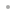FeaturesExcelLinkMathematicaLinkWhat's New in Version 3Who's It For?ExamplesUser's Manual and Reference Guide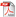Buy Online### Commands

Intuitive user-interface components allow you to communicate with Mathematica from Excel.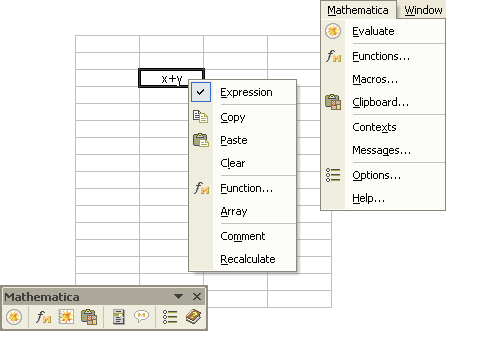Interact via the Mathematica toolbar, the Mathematica context menu and the Mathematica menu.

### Functions

If you are unsure of the name of a Mathematica function or how to use it, or want to explore the functions Mathematica has to offer, the Mathematica function wizard provides an easy way to access this information directly in Excel.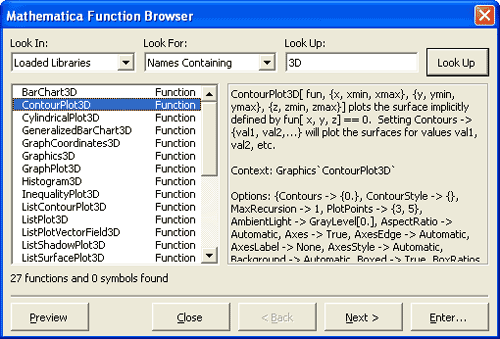Find and learn about functions using the Mathematica function wizard.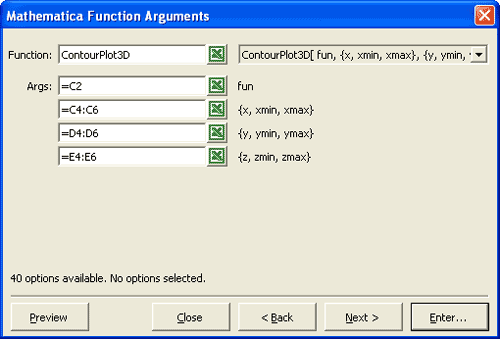Specify arguments using the Mathematica function wizard.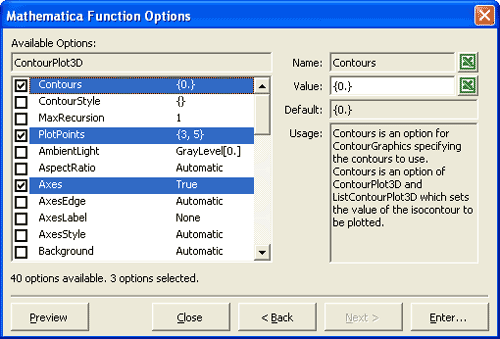Specify options using the Mathematica function wizard.

### Macros

Mathematica Link for Excel makes it easy to create Excel macros that call Mathematica.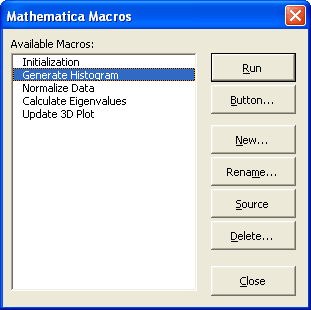Manage your macros using the Mathematica macros dialog.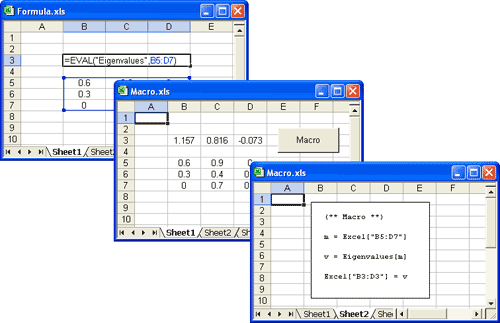Create spreadsheet formulas and macros that call Mathematica.

### Clipboard

An intelligent clipboard makes it easy to eliminate copy-and-paste errors and to clean up data.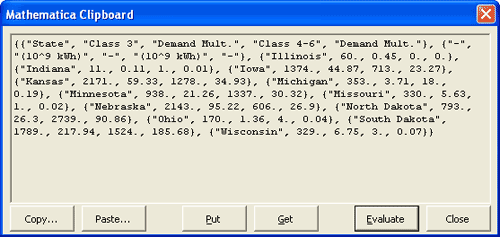Copy data using the interactive Mathematica clipboard window.